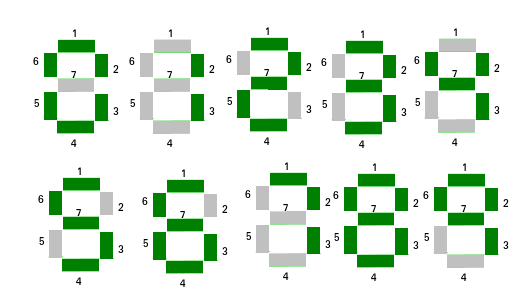GeeksforGeeks App
Open AppBrowser
Continue

## Related Articles

• Write an Interview Experience
• Mathematical Algorithms

# Find element using minimum segments in Seven Segment Display

A Seven Segment Display can be used to display numbers. Given an array of n natural numbers. The task is to find the number in the array which is using minimum segments to display number. If multiple numbers have a minimum number of segments, output the number having the smallest index.Examples :

```Input : arr[] = { 1, 2, 3, 4, 5 }.
Output : 1
1 uses on 2 segments to display.

Input : arr[] = { 489, 206, 745, 123, 756 }.
Output : 745```
Recommended Practice

Precompute the number of segment used by digits from 0 to 9 and store it. Now for each element of the array sum the number of segment used by each digit. Then find the element which is using the minimum number of segments.

```The number of segment used by digit:
0 -> 6
1 -> 2
2 -> 5
3 -> 5
4 -> 4
5 -> 5
6 -> 6
7 -> 3
8 -> 7
9 -> 6```

Below is the implementation of this approach:

## C++

 `// C++ program to find minimum number of segments``// required``#include``using` `namespace` `std;` `// Precomputed values of segment used by digit 0 to 9.``const` `int` `seg = { 6, 2, 5, 5, 4, 5, 6, 3, 7, 6};` `// Return the number of segments used by x.``int` `computeSegment(``int` `x)``{``    ``if` `(x == 0)``        ``return` `seg;` `    ``int` `count = 0;` `    ``// Finding sum of the segment used by``    ``// each digit of a number.``    ``while` `(x)``    ``{``        ``count += seg[x%10];``        ``x /= 10;``    ``}` `    ``return` `count;``}` `int` `elementMinSegment(``int` `arr[], ``int` `n)``{``    ``// Initialising the minimum segment and minimum``    ``// number index.``    ``int` `minseg = computeSegment(arr);``    ``int` `minindex = 0;` `    ``// Finding and comparing segment used``    ``// by each number arr[i].``    ``for` `(``int` `i = 1; i < n; i++)``    ``{``        ``int` `temp = computeSegment(arr[i]);` `        ``// If arr[i] used less segment then update``        ``// minimum segment and minimum number.``        ``if` `(temp < minseg)``        ``{``            ``minseg   = temp;``            ``minindex = i;``        ``}``    ``}` `    ``return` `arr[minindex];``}` `// Driven Program``int` `main()``{``    ``int` `arr[] = {489, 206, 745, 123, 756};``    ``int` `n = ``sizeof``(arr)/``sizeof``(arr);``    ``cout << elementMinSegment(arr, n) << endl;``    ``return` `0;``}`

## Java

 `// Java program to find minimum``// number of segments required``import` `java.io.*;` `class` `GFG {``    ` `// Precomputed values of segment``// used by digit 0 to 9.``static` `int` `[]seg = { ``6``, ``2``, ``5``, ``5``, ``4``, ``5``, ``6``, ``3``, ``7``, ``6``};` `// Return the number of segments used by x.``static` `int` `computeSegment(``int` `x)``{``    ``if` `(x == ``0``)``        ``return` `seg[``0``];` `    ``int` `count = ``0``;` `    ``// Finding sum of the segment used by``    ``// each digit of a number.``    ``while` `(x > ``0``)``    ``{``        ``count += seg[x % ``10``];``        ``x /= ``10``;``    ``}` `    ``return` `count;``}` `static` `int` `elementMinSegment(``int` `[]arr, ``int` `n)``{``    ``// Initialising the minimum segment``    ``// and minimum number index.``    ``int` `minseg = computeSegment(arr[``0``]);``    ``int` `minindex = ``0``;` `    ``// Finding and comparing segment used``    ``// by each number arr[i].``    ``for` `(``int` `i = ``1``; i < n; i++)``    ``{``        ``int` `temp = computeSegment(arr[i]);` `        ``// If arr[i] used less segment then update``        ``// minimum segment and minimum number.``        ``if` `(temp < minseg)``        ``{``            ``minseg = temp;``            ``minindex = i;``        ``}``    ``}` `    ``return` `arr[minindex];``}` `    ``// Driver program``    ``static` `public` `void` `main (String[] args)``    ``{``       ``int` `[]arr = {``489``, ``206``, ``745``, ``123``, ``756``};``       ``int` `n = arr.length;``       ``System.out.println(elementMinSegment(arr, n));``    ``}``}` `//This code is contributed by vt_m.`

## Python3

 `# Python implementation of``# the above approach` `# Precomputed values of segment``# used by digit 0 to 9.``seg ``=` `[``6``, ``2``, ``5``, ``5``, ``4``,``       ``5``, ``6``, ``3``, ``7``, ``6``]` `# Return the number of``# segments used by x.``def` `computeSegment(x):``    ``if``(x ``=``=` `0``):``        ``return` `seg[``0``]` `    ``count ``=` `0` `    ``# Finding sum of the segment``    ``# used by each digit of a number.``    ``while``(x):``        ``count ``+``=` `seg[x ``%` `10``]``        ``x ``=` `x ``/``/` `10` `    ``return` `count` `# function to return minimum sum index``def` `elementMinSegment(arr, n):` `    ``# Initialising the minimum``    ``# segment and minimum number index.``    ``minseg ``=` `computeSegment(arr[``0``])``    ``minindex ``=` `0` `    ``# Finding and comparing segment``    ``# used by each number arr[i].``    ``for` `i ``in` `range``(``1``, n):``        ``temp ``=` `computeSegment(arr[i])` `        ``# If arr[i] used less segment``        ``# then update minimum segment``        ``# and minimum number.``        ``if``(temp < minseg):` `            ``minseg ``=` `temp``            ``minindex ``=` `i` `    ``return` `arr[minindex]` `# Driver Code``arr ``=` `[``489``, ``206``, ``745``, ``123``, ``756``]``n ``=` `len``(arr)` `# function print required answer``print``(elementMinSegment(arr, n))` `# This code is contributed by``# Sanjit_Prasad`

## C#

 `// C# program to find minimum``// number of segments required``using` `System;` `class` `GFG{``    ` `// Precomputed values of segment``// used by digit 0 to 9.``static` `int` `[]seg = ``new` `int``{ 6, 2, 5, 5, 4,``                               ``5, 6, 3, 7, 6};` `// Return the number of segments used by x.``static` `int` `computeSegment(``int` `x)``{``    ``if` `(x == 0)``        ``return` `seg;` `    ``int` `count = 0;` `    ``// Finding sum of the segment used by``    ``// each digit of a number.``    ``while` `(x > 0)``    ``{``        ``count += seg[x % 10];``        ``x /= 10;``    ``}` `    ``return` `count;``}` `static` `int` `elementMinSegment(``int` `[]arr, ``int` `n)``{``    ``// Initialising the minimum segment``    ``// and minimum number index.``    ``int` `minseg = computeSegment(arr);``    ``int` `minindex = 0;` `    ``// Finding and comparing segment used``    ``// by each number arr[i].``    ``for` `(``int` `i = 1; i < n; i++)``    ``{``        ``int` `temp = computeSegment(arr[i]);` `        ``// If arr[i] used less segment then update``        ``// minimum segment and minimum number.``        ``if` `(temp < minseg)``        ``{``            ``minseg = temp;``            ``minindex = i;``        ``}``    ``}` `    ``return` `arr[minindex];``}` `    ``// Driver program``    ``static` `public` `void` `Main()``    ``{``       ``int` `[]arr = {489, 206, 745, 123, 756};``       ``int` `n = arr.Length;``       ``Console.WriteLine(elementMinSegment(arr, n));``    ``}``}` `//This code is contributed by vt_m.`

## PHP

 ``

## Javascript

 ``

Output

`745`

Time Complexity: O(n * log10n)
Auxiliary Space: O(10)

This article is contributed by Anuj Chauhan. If you like GeeksforGeeks and would like to contribute, you can also write an article using write.geeksforgeeks.org or mail your article to review-team@geeksforgeeks.org. See your article appearing on the GeeksforGeeks main page and help other Geeks.

My Personal Notes arrow_drop_up